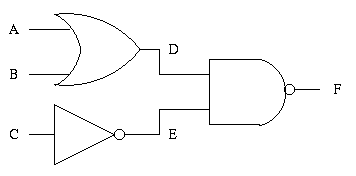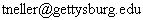CS 371 Introduction to Artificial Intelligence Homework #4

Due: Tuesday 9/26
1. Truth tables, validity, and satisfiability: R&N 6.1
2. Equivalence rules: R&N 6.3 a,c,e,g

3. Abbreviate Smoke, Fire, etc. as S, F, etc.
Hint: Simplify expressions (and reduce truth table work) using equivalence rules.
4. Propositional reasoning: R&N 6.5

5. Use the following propositional symbols to reduce your writing:
• P: The unicorn is mythical.
• Q: The unicorn is immortal.
• R: The unicorn is a mammal.
• S: The unicorn has a horn.
• T: The unicorn is magical.
If the proposition can be proven, provide a step-by-step proof. If it cannot be proven, demonstrate this by providing an appropriate model.
Hint: A simple proof method is refutation (also known as proof by contradiction, see R&N p. 280).
Another hint: You can use the equivalence rules of Exercise 6.2 to construct equivalence sentences helpful to your proof. Each equivalence can be written as a conjunction of two implications, each of which can be used with modus ponens. (Contraposition may be especially useful depending on your approach.)
6. Models: R&N 6.7
7. implies, entails, derives:
1. Describe the difference between "implies", "entails", and "derives".
2. What word describes an inference procedure that entails what it derives?
3. What word describes an inference procedure that derives what it entails?
8. Using GSAT:
1. Convert the following sentences into conjunctive normal form (CNF):
1. P implies Q
2. (not P) implies ((not Q) and R)
3. (Q or R) implies S
4. S implies T
2. Use GSAT to test whether or not this CNF KB does not entail:
1. P
2. S
3. T
3. Could GSAT be used to prove that propositional logic formulas are entailed by a CNF KB?  Why (and how) or why not?

4. Be sure to include all input and output files in your README file.
9. Practical application: Boolean satisfiability can be used to generate test patterns for a single "stuck-at" fault in combinational circuits. First read section 2.1 of T. Larrabee. Test Pattern Generation Using Boolean Satisfiability. In IEEE Transactions on Computer-Aided Design, pages 4-15, Jan, 1992.
1. Derive a CNF formula which, when satisfied provides a test pattern to check whether D is stuck at 0 in the following circuit:
2.3. Use GSAT to find a satisfying assignment to the above formula. Hand in your input and output file. How would you then use this satisfying assignment to test for the fault?
10. GSAT and 3CNF problem difficulty: R&N 6.15 a,b,d,f. See the GSAT How-To guide for information about the gtime script.

(c) 2000 Todd Neller